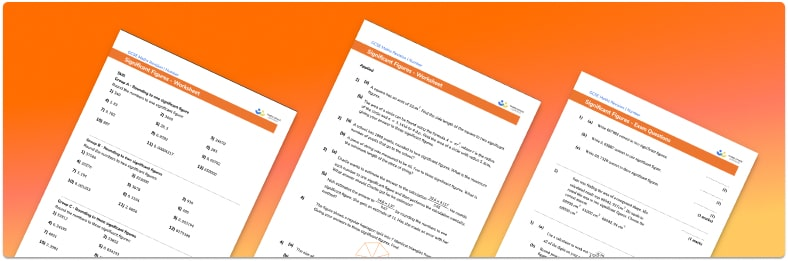# Significant Figures Worksheet• Section 1 of the significant figures worksheet contains 36 skills-based questions, in 3 groups to support differentiation
• Section 2 contains 4 applied significant figures questions with a mix of worded problems and deeper problem solving questions
• Section 3 contains 4 foundation and higher level GCSE exam style significant figures questions
• Answers and a mark scheme for all significant figures questions
• Follows variation theory with plenty of opportunities for students to work independently at their own level
• All questions created by fully qualified expert secondary maths teachers
• Suitable for GCSE maths revision for AQA, OCR and Edexcel exam boards

• This field is for validation purposes and should be left unchanged.

You can unsubscribe at any time (each email we send will contain an easy way to unsubscribe). To find out more about how we use your data, see our privacy policy.

### Significant figures at a glance

Significant figures (also referred to as sig figs or sf) are digits which contribute to the value of a number. We start counting significant figures from the first non-zero digit. For example, in the number 23.0961, the first significant figure is 2, the second significant figure is 3, the third significant figure is 0 and so on.

We can round to a given number of significant figures in the same way we can round to a given number of decimal places. For example, if we want to round to 3 significant figures, we start by identifying the third significant digit (this could come before or after a decimal point). We then look at the next digit, if this is 5 or greater we round up and if it is 4 or less we round down.

As an alternative to rounding numbers to the nearest significant figure, we can round to other degrees of accuracy such as the nearest 10, nearest whole number or nearest hundredth.

Looking forward, students can then progress to additional number worksheets, for example aorFor more teaching and learning support on Number our GCSE maths lessons provide step by step support for all GCSE maths concepts.

## Do you have KS4 students who need more focused attention to succeed at GCSE?There will be students in your class who require individual attention to help them succeed in their maths GCSEs. In a class of 30, it’s not always easy to provide.

Help your students feel confident with exam-style questions and the strategies they’ll need to answer them correctly with our dedicated GCSE maths revision programme.

Lessons are selected to provide support where each student needs it most, and specially-trained GCSE maths tutors adapt the pitch and pace of each lesson. This ensures a personalised revision programme that raises grades and boosts confidence.

Find out more